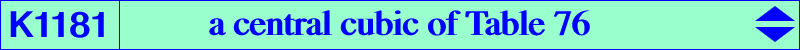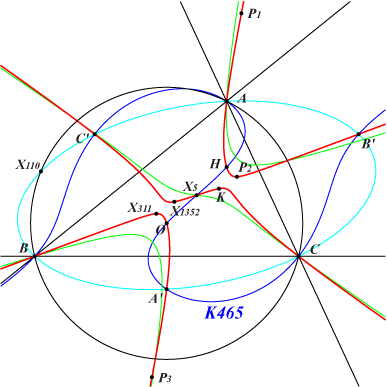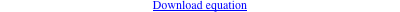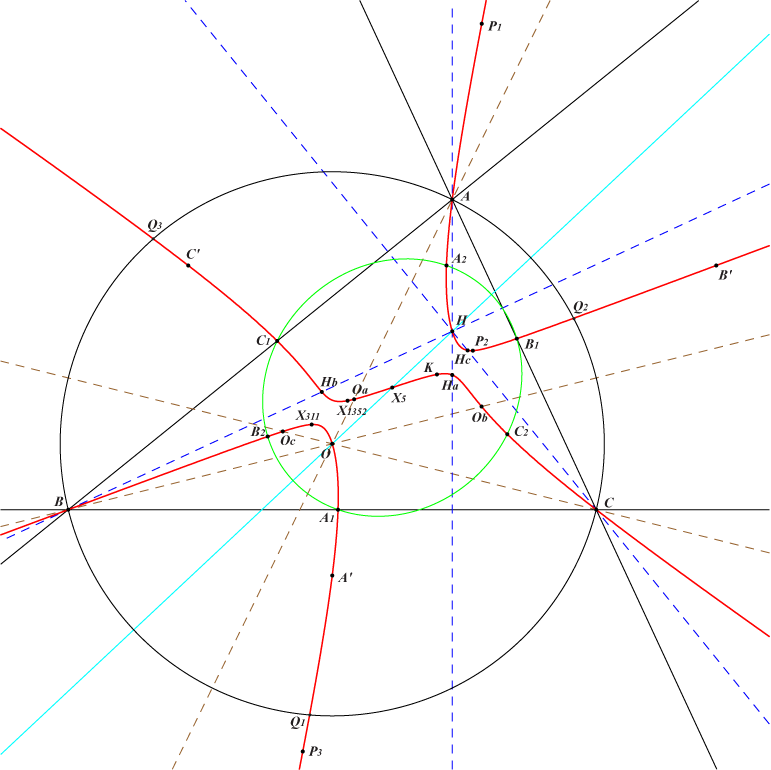too complicated to be written here. Click on the link to download a text file.X(3), X(4), X(5), X(6), X(311), X(1352) other points below Geometric properties :K1181 is a central cubic with center X(5), a member of the pencil mentioned in Table 76, which contains the circular cubic K465 and the stelloid K026. Points on K1181 : • A', B', C' : centers of the Johnson circles, reflections of A, B, C in X(5). These points lie on the perpendicular bisectors of ABC. • A1, B1, C1 : on the sidelines of ABC and on the lines passing through X(1352) and A', B', C' respectively. • A2, B2, C2 : reflections of A1, B1, C1 in X(5). These points are the "last" points on the symmedians of ABC. • infinite points of pK(X6, X5012), pK(X39, X3), pK(X27371, X4), pK(X51, X6), pK(X216, X22). More generally, for every Ω on psK(X5 x X39, X2, X6), there is a pK with pole Ω meeting the line at infinity at the same points as K1181. The complement of the corresponding pivot lies on psK(X5 x X39, X2, X3). • Q1, Q2, Q3 : on the circumcircle and on pK(X6, Q) with Q on the lines {4,69}, {5,49}, {52,2888}. • P1, P2, P3 : third points of K1181 on the lines {3,1352}, {3,6}, {4,6} respectively. P1, P3 and P2, X(311) are symmetric about X(5). • Ha, Hb, Hc : third points of K1181 on the altitudes. Ha lies on the line A1, P2. • Oa, Ob, Oc : third points of K1181 on the cevian lines of O. Oa lies on the line A1, P3. Note that the lines HbOc and HcOb meet at A2. Analogous properties are obtained with B2, C2. • Ha', Hb', Hc' : reflections of Ha, Hb, Hc in X(5). These points are the "last" points on the perpendicular bisectors of ABC. • Oa', Ob', Oc' : reflections of Oa, Ob, Oc in X(5). • Q1', Q2', Q3' : reflections of Q1, Q2, Q3 in X(5). These points lie on the circle with center H, radius R, passing through X(265). *** P1 = (a^2-b^2-c^2) (a^4+b^4-c^4)(a^4-b^4+c^4) (a^2 b^2-b^4+a^2 c^2+2 b^2 c^2-c^4) : : , SEARCH = 1.34068367609858 P2 = a^2 (a^2 b^2-b^4+a^2 c^2+2 b^2 c^2-c^4) (a^4 b^2-2 a^2 b^4+b^6+a^4 c^2-3 a^2 b^2 c^2-2 a^2 c^4+c^6) : : , SEARCH = -1.06939666061101 P3 = (-a^2+b^2-c^2) (a^2+b^2-c^2) (-a^2 b^2+b^4-a^2 c^2-2 b^2 c^2+c^4) (-a^10+a^8 b^2+2 a^6 b^4-2 a^4 b^6-a^2 b^8+b^10+a^8 c^2+4 a^6 b^2 c^2-4 a^4 b^4 c^2-b^8 c^2+2 a^6 c^4-4 a^4 b^2 c^4+2 a^2 b^4 c^4-2 a^4 c^6-a^2 c^8-b^2 c^8+c^10) : : , SEARCH = -0.234940192828766 Q = a^10-2 a^8 b^2+a^6 b^4-a^4 b^6+2 a^2 b^8-b^10-2 a^8 c^2+3 a^6 b^2 c^2-3 a^4 b^4 c^2-a^2 b^6 c^2+3 b^8 c^2+a^6 c^4-3 a^4 b^2 c^4-2 a^2 b^4 c^4-2 b^6 c^4-a^4 c^6-a^2 b^2 c^6-2 b^4 c^6+2 a^2 c^8+3 b^2 c^8-c^10 : : , SEARCH = 1.16553879304391 These points are now X(41168), X(41169), X(41170), X(41171) in ETC.